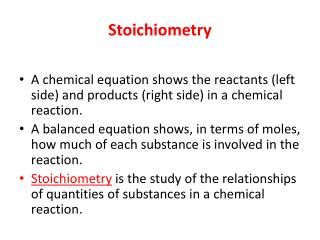Download PresentationStoichiometry

# Stoichiometry - PowerPoint PPT PresentationDownload Presentation## Stoichiometry

- - - - - - - - - - - - - - - - - - - - - - - - - - - E N D - - - - - - - - - - - - - - - - - - - - - - - - - - -
##### Presentation Transcript

1. Stoichiometry • A chemical equation shows the reactants (left side) and products (right side) in a chemical reaction. • A balanced equation shows, in terms of moles, how much of each substance is involved in the reaction. • Stoichiometryis the study of the relationships of quantities of substances in a chemical reaction.

2. FeCl3 (aq) + 3NaOH (aq) → Fe(OH)3 (s) + 3NaCl (aq) one mole of iron(III) chloride reacts with three moles of sodium hydroxide to produce one mole of iron(III) hydroxide and three moles of sodium chloride. Several mole ratio fractions are possible:

3. FeCl3 (aq) + 3NaOH (aq) → Fe(OH)3 (s) + 3NaCl (aq)EXAMPLE: What mass of sodium hydroxide is needed to react completely with 10.0 g of iron(III) chloride? Convert Mass  Moles Compare Moles of each Convert Moles  Molar Mass 7.39g NaOH is needed to react completely with 10.0g of FeCl3

4. You TryFeCl3 (aq) + 3NaOH (aq) → Fe(OH)3 (s) + 3NaCl (aq) • EXAMPLE: What mass of iron(III) hydroxide will be formed from 10.0 g of iron(III) chloride? Convert Mass  Moles Compare Moles of each Convert Moles  Molar Mass • 6.58g Fe(OH)3is needed to react completely with 10.0g of FeCl3

5. Limiting Reagent Problems • You are given amounts of both reactants and asked to find the amount of product formed. • Usually the amount of one of the reactants given is in excess • The yield (amount) of product is determined by the reactant present in thesmaller amount: the limiting reagent. • First you decide which is the limiting reagent then calculate the amount of product formed.

6. FeCl3(aq) + 3NaOH (aq) → Fe(OH)3 (s) + 3NaCl (aq) • What mass of iron(III) hydroxide will be formed if a solution containing 10.0 g of iron(III) chloride is mixed with a solution containing 10.0 g of sodium hydroxide? • STEP 1 – Find Limiting reagent = 0.0616 mol FeCl3 given = 0.250 mol NaOHgiven

7. Take either of these moles of reactants and calculate how much of the other reactant would be needed to react completely with it: Let us take moles of FeCl3 0.0616 mol FeCl3 given 0.250 mol NaOHgiven • Since MORE NaOH is given than needed it is in EXCESS • FeCl3 is the limiting reagent

8. Step 2- Calculate amount of product formed